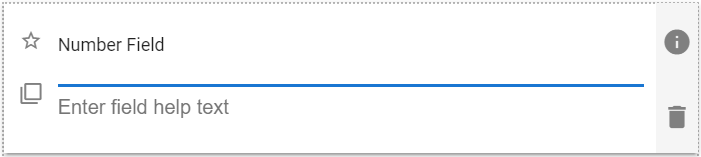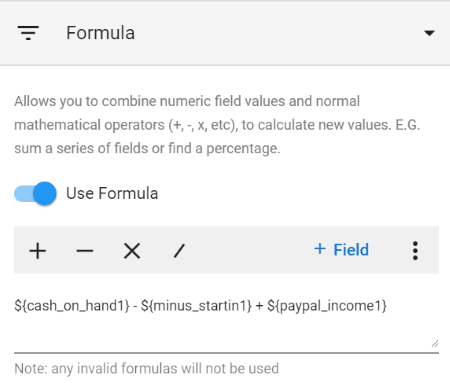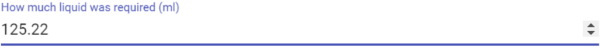1. Home
2. Forms
3. Form Fields & Components
4. Form Fields & Components: Number

# Form Fields & Components: Number

Contents

A number field. In use, the field will display up/down arrow controls at its far right side. Using the arrow controls will change the number to the next higher or lower integer value.

 The default look of the Number component## Number Field Properties

 Setting Description Basic Tab / General Settings Display Name Default Value Field Help Text Required See General Field Features Basic Tab / Validation Section Minimum Allowed Value Enable to set a minimum field value. Displays the following two fields when enabled: Min Value Enter the desired minimum field value. Message to show user Enter the text to display when the user entered value is smaller than the Min Value. Maximum Allowed Value Enable to set a maximum field value. Displays the following two fields when enabled: Max Value Enter the desired maximum field value. Message to show user Enter the text to display when the user entered value is larger than the Max Value. Basic Tab / Format Section Percentage Toggle Enable to define the number as a percentage value: i.e. 0.12 displays as 12%. Decimal Places Enter the desired number of decimal places. Advanced Tab / Formula Tab – See below Advanced Tab / Conditional Visibility – See Conditional Visibility

## Using Formulas

 Formula SettingsYou create the desired formula by combining fields and the four basic mathematical operators of + (addition), – (subtraction), X (multiplication) and / (division). You can also directly type parenthesis brackets “( )” around parts of the formula to ensure it is calculated in the correct way. (Normal mathematical rules apply.)

 Operator Description + Addition: Adds ‘ + ‘ to the formula at the current cursor position. (A space character is included either side of the + symbol.) – Subtraction: Adds ‘ – ‘ to the formula at the current cursor position. (A space character is included either side of the – symbol.) x Multiplication: Adds ‘ x ‘ to the formula at the current cursor position. (A space character is included either side of the x symbol.) / Division: Adds ‘ / ‘ to the formula at the current cursor position. (A space character is included either side of the / symbol.) + Field Briefly displays a field selection drop-down to allow you to select the desired field. The drop-down also has search functionality. Once the desired field is selected, the short code for that field is added to the formula, with the syntax \${field_short_code}. In the formula above, \${cash_on_hand1} is an example of a field.

If the formula is not valid the field will be highlight in red, and ‘Invalid Formula’ is displayed.

## Number Field Usage Example

 Number usage example## Demonstration Video

The following video outlines creating number fields:

Updated on May 11, 2021